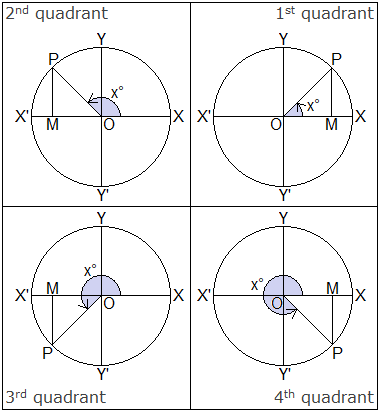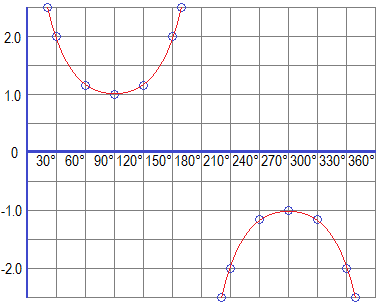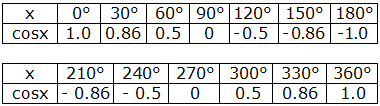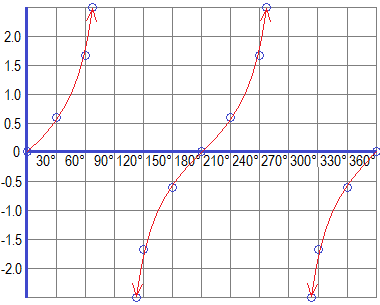# Graphs of Trigonometric Functions## Graphs of Trigonometric Functions

To each angle x, there corresponds a unique value for the trigonometric ratios and hence the trigonometric ratios such as sinx, cosx, tanx including the reciprocal ratios are the functions of the angle x.

********************

10 Math Problems officially announces the release of Quick Math Solver, an Android App on the Google Play Store for students around the world.

********************

A function can be represented in various ways. One of the important ways by which a function can be represented is the graph. In a trigonometric function, the variable is the angle. For different values of the angle, the values of the trigonometric ratios will be different.

To draw the graph of a trigonometric function, we take the angle x along the X-axis and the value of trigonometric ratio sinx, cosx, tanx, etc. along the Y-axis. Taking different values of the angle as the x-coordinates, and the corresponding values of the trigonometric ratio as y-coordinates, we plot the points (x, sinx), etc. on the plane with rectangular axes. Then we join these points freely to get the required graph of the trigonometric function.

Consider a circle with radius r placed in standard position. Let a revolving line OP start from OX and trace out an angle XOP = x°. Draw perpendicular PM from P to the X-axis.OP revolves from OX to OY, x varies from 0° to 90°, MP varies from 0 to r, MP is positive, OM varies from r to 0, and OM is positive.

OP revolves from OY to OX’, x varies from 90° to 180°, MP varies from r to 0, MP is positive, OM varies from 0 to – r and OM is negative.

OP revolves from OX’ to OY’, x varies from 180° to 270°, MP varies from 0 to – r, MP is negative, OM varies from – r to 0 and OM is negative.

OP revolves from OY’ to OX, x varies from 270° to 360°, MP varies from – r to 0, MP is negative, OM varies from 0 to r, OM is positive.

### Graph of Sine Function (Sine Graph)

The sine function is defined by y = sinx = MP/OP = MP/r.

When x varies from 0° to 90°, y = sinx varies from 0 to 1 because MP varies from 0 to r. When x varies from 90° to 180°, y = sinx varies from 1 to 0 because MP varies from r to 0. When x varies from 180° to 270°, y = sinx varies from 0 to – 1 because MP varies from 0 to – r. When x varies from 270° to 360°, y = sinx varies from – 1 to 0.

Some standard values of x and the corresponding values of sinx are given in the table below:Plotting these values in the graph paper, we get the following graph of the sine function (Sine Graph).If we study this sine curve, we will get the following facts:

(i)    y = sinx attains the maximum value at 90° and minimum value at 270°. The maximum and minimum value of sinx are 1 and – 1 respectively. So y = sinx oscillates in the limit – 1 to 1.

(ii)   y = sinx has positive values in the first and second quadrants where the graph is above the X-axis and negative values in the third and fourth quadrants where the graph is below X-axis.

### Graph of Cosecant Function (Cosecant Graph)

Similarly, we can have the table of trigonometric values and graph of the reciprocal of sine function i.e cosecant function (y = cosecx) as given below:Plotting these values in the graph paper, we get the following graph of the cosecant function (Cosecant Graph).### Graph of Cosine Function (Cosine Graph)

The cosine function is defined by y = cosx = OM/OP = OM/r.

When x increases from 0° to 90°, cosx decreases from 1 to 0 as OM decreases from r to 0. When x increases from 90° to 180°, cosx decreases from 0 to – 1 because OM changes 0 to – r. When x  changes from 180° to 270°, cosx changes from – 1 to 0. Similarly, when x increases from 270° to 360°, cosx increases from 0 to 1.

Some standard values of x and the corresponding values of cosx are given in the table below:Plotting these values in the graph paper, we get the following graph of the cosine function (Cosine Graph).If we study this cosine curve, we will get the following facts:

(i)    y = cosx attains the maximum value at x = 0° and 360° and minimum value at 180°. The maximum and minimum value of cosx are 1 and – 1 respectively. So y = cosx oscillates in the limit – 1 to 1.

(ii)   y = cosx has positive values in the first and fourth quadrants where the graph is above the X-axis and negative values in the second and third quadrants where the graph is below X-axis.

### Graph of Secant Function (Secant Graph)

Similarly, we can have the table of trigonometric values and graph of the reciprocal of cosine function i.e secant function (y = secx) as given below:Plotting these values in the graph paper, we get the following graph of the secant function (Secant Graph).### Graph of Tangent Function (Tangent Graph)

The tangent function is defined by y = tanx = MP/OM

When x increases from 0° to 90°, tanx increases from 0 to ∞ (infinity) because MP changes from 0 to r and OM changes from r to 0. When x increases from 90° to 180°, tanx changes from - ∞ to 0 because MP changes from r to 0 and OM changes from 0 to – r. When x increases from 180° to 270°, tanx changes from 0 to ∞ because MP changes from 0 to – r, and OM changes from – r to 0. When x increases from 270° to 360°, tanx changes from - ∞ to 0 because MP changes from – r to 0 and OM changes from 0 to r.

Some standard values of x and the corresponding values of tanx are given in the table below:Plotting these values in the graph paper, we get the following graph of the tangent function (Tangent Graph).y = tanx has positive values in the first and third quadrants where the graph is above X-axis and negative values in the second and fourth quadrants where the graph is below X-axis. The maximum and minimum values of tanx cannot be defined.

### Graph of Cotangent Function (Cotangent Graph)

Similarly, we can have the table of trigonometric values and graph of the reciprocal of tangent function i.e cotangent function (y = cotx) as given below:Plotting these values in the graph paper, we get the following graph of the cotangent function (Cotangent Graph).#### Do you have any questions regarding the graph of a trigonometric function?

You can ask your questions or problems here, in the comment section below.